Introduction to forensic engineering

Start this free course now. Just create an account and sign in. Enrol and complete the course for a free statement of participation or digital badge if available.

Free course

# 3.5 Mechanical testing

Mechanical failure is a common failure mode for a wide variety of products. It therefore makes sense to conduct mechanical tests on the product to indicate the weak points in its design. It is important the product is tested under identical, or near identical, conditions to those expected in service. So, the load or stress system to which the product will be exposed should be analysed closely.

A common set of different types of stress is shown in Table 5, together with a set of typical examples. Most are simple, such as torsion, which mainly puts the sample under shear. But a bending stress is a combination that places the convex surface in tension and the concave surface in compression. In bending, there may also be a shear component if the section is thick enough.

Choosing the material of construction is an important part of the design phase. Suppliers usually make a material available in sheet form, from which samples can be taken for simple tensile testing to give values for strength and stiffness. What such tests do not reveal however, is the sensitivity of many materials to the effects of product geometry.

## Table 5: Stress types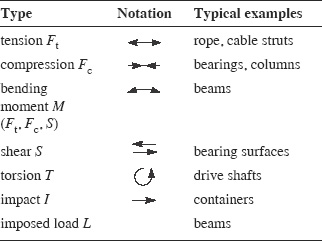## 3.5.1 Stress concentrations

There are many reasons why materials in products fall short of the strength predicted from the simple tensile test. One of the most important reasons is the way applied stresses can be concentrated at particular points in products. Such points are known as stress concentrators, and their effects can be predicted using relatively simple mechanical models.

Figure 38 visualises the stress lines in loaded samples. Owing to the restrictions of product geometry, the lines are squeezed past the tips of such features as cracks. The net result is that a stress concentration exists at the tip of a crack. Sharp corners in products are the source of similar stress concentration.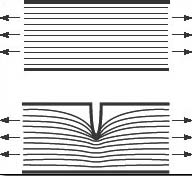Figure 38: Flat bar with imaginary lines of force created by tensile load along its axis. At top, the lines are parallel and regular. At bottom, the notch causes the lines of force to distort and to focus at the tip of the notch, so creating a stress concentration

Perhaps less easy to appreciate are the effects of internal voids or internal cut-aways, but these too can cause serious stress concentrations, as Box 14 describes.

### Box 14 Stress concentrations

Stress concentrations can be modelled mathematically by several different methods. The stress concentration at a notch tip in an elastic material can be modelled relatively easily. The stress, sigma (symbol σ), at the notch tip, is given by the equation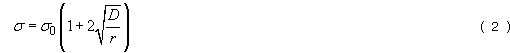where σ0 is the uniform stress applied to the material sample; D is the depth of the notch from a free surface; and r is the radius of curvature at the notch tip.

In the centre of Figure 39 are two examples where the above equation can be used. At the left and right of Figure 39, there are other features that will concentrate stress. If there was a cylindrical hole through a flat bar the stress would be three times greater because D = r (two semi-circular notches side-by-side). A spherical void would produce a stress two times greater because the stress is distributed over the larger internal surface area of the void.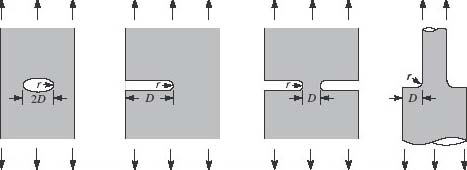Figure 39: Examples of stress concentration: a hole, a notch, two opposing notches, a narrowing section with a chamfer

The design engineer can use standard reference works to estimate the effect of other stress raisers. A textbook example is shown in Figure 40, where a sketch and a graph represent a notch in a plate subject to bending. Figure 40 is versatile because it can be used for a variety of design configurations. Finite element analysis programs are also available, and are based on standard formulae.

It is possible to model particular configurations physically. The method involves constructing a model in birefringent plastic such as polyurethane or polycarbonate. When it is strained under the expected conditions and polarised light is shone through it, a fringe pattern shows the stress concentrations.

You need to be aware of escalation when stress concentrations interact. The net effect is the product of the individual factors, so it is more serious than expected. Thus, a sharp crack at a notch tip is more serious than either alone. Similarly, a circular groove inside another circular groove produces a net effect of nine times greater because the net effect of the two stress raisers is the product of the effect of each individual stress concentration.

Stress concentrations are ameliorated in many tough materials because yielding occurs at the critical zone, especially if the sample is loaded slowly. However, it is not always possible to predict the effect, so stress raisers due to shape should be ameliorated wherever possible. Where the material is known to be brittle, or where fatigue is possible – even in a tough material – every attempt should be made at the design stage to reduce stress raisers.

The effect is normally described in terms of the stress concentration factor Kt. This is simply the ratio of the nominal imposed stress σ0 and the actual stress σ at the feature in question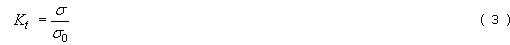For any Kt value greater than unity, the actual stress at the stress concentrator will be greater than the nominal imposed stress, and if the actual stress exceeds the strength of the material, the sample will fail. If a brittle crack is initiated at a stress raiser, the net section area becomes smaller. The crack therefore accelerates until the stress is relieved. Again, depending on sample geometry, it could result in the product breaking into two, or possibly more, parts with a consequent loss of function.

### SAQ 10

What points in the solid thermoplastic products in Figure 41 are most vulnerable to mechanical failure by cracking? Estimate the stress concentrations at those points under the loading condition shown by the grey arrows. For (a) r = 0.25 mm, t = 2.5 mm; for (b) r = 0.4 mm, t = 8 mm; for (c) r = 0.1 mm, t = 2 mm, D 0 = 22 mm, D 1 = 30 mm.

Use Box 14 as a guide. How would you reduce the weakness in the products?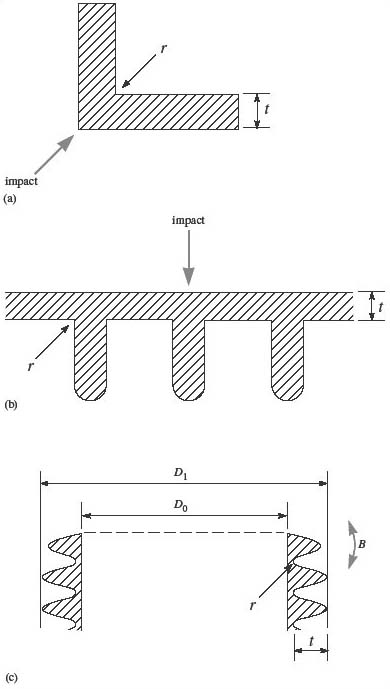Figure 41: Sections with stress raisers: (a) corner in right angle; (b) ribbed plate; (c) tube with screw thread

In Figure 41(a), the weakest part of the product is the internal corner. The stress concentration can be estimated by using Figure 40, in Box 14, with the given dimensions.

You can use the diagonal distance between the two corners for d and then you can calculate r/d. Pythagoras' theorem or trigonometry will give you d.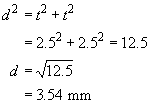So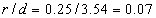Reading from the curve of Figure 40 gives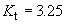So an impact on the corner will cause a stress about 3.25 times the nominal applied stress.

In Figure 41(b), an impact against the flat side of the ribbed plate will produce a bending load on the rib corners, so they represent the weakest parts of the structure. Using the same method as above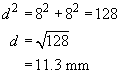So

r/d = 0.4/11.3 = 0.035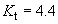In Figure 41(c), the thread root is the weakest part of the system, and the part will fracture from here when a bending load is applied. The stress concentration can be estimated as before using Figure 40.

The distance from the root to the inner surface is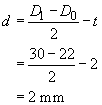So

r/d = 0.1/2 = 0.05

Kt = 3.75

### SAQ 11

Figure 42 shows a simple box made from rigid plastic filled with liquid. Assume when dropped, it will suffer impact on one of the corners. Knowing the radius of curvature r at the inner corners is 0.1 mm, and the wall t is 4 mm thick, estimate the stress concentration at the centre of each corner, and as a result, predict which corner is most likely to fail. What would be the effect if the radius were to be increased to 1 mm? What other steps could be taken to improve product performance? Refer to Box 14 for help with the problem.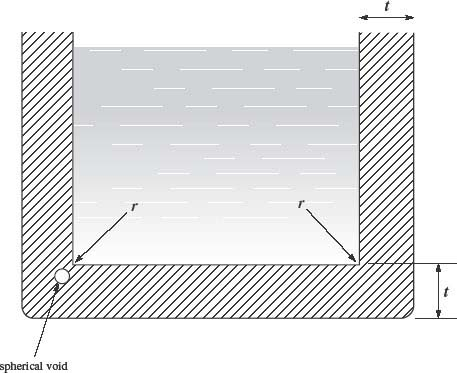Figure 42: Cross section of plastic box containing liquid

The stresses can be evaluated using Figure 40, Box 14. For the right-hand corner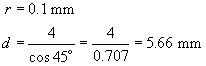So

r/d = 0.1/5.66 = 0.018

Reading from Figure 40 for an r/d value of 0.02 gives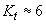So this corner will experience a stress concentration of about six times the applied stress.

The situation for the left-hand corner is more complicated, because there is a spherical void near the inner corner. The net stress raising effect will be the product of each stress raiser: the inner corner and the void. Using the multiplier 2 from Box 14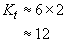So failure will probably occur at the left-hand corner.

The effect of increasing the corner radius ten times to 1 mm can simply be evaluated in the same way, using an r/d value of 0.18. Reading from Figure 40

Kt = 2.2

This represents the stress concentration for the right-hand corner; that for the opposite corner is twice this, giving Kt = 4.4. The relative situation between the two corners is unchanged, although the severity is much reduced, by about a factor of three. Note that a further increase of radius would produce diminishing returns as the curve flattens out at higher r/d values.

An alternative design strategy might involve changing the depth of material at the corner by either increasing the overall wall thickness or thickening the corners locally. The origin of the void may be a result of poor quality material or poor manufacturing, so attention here could solve that problem.